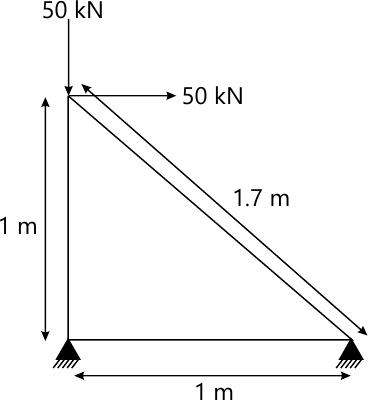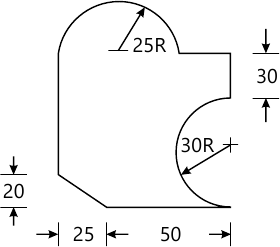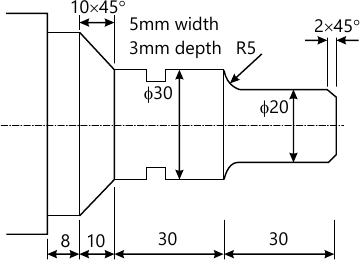SPPU Mechanical Engineering (Semester 7)
June 2015
Total marks: --
Total time: --
INSTRUCTIONS
(1) Assume appropriate data and state your reasons
(2) Marks are given to the right of every question
(3) Draw neat diagrams wherever necessary

Answer any one question from Q1 and Q2
1 (a) What is Open GL? Explain its use in Software Customization.
6 M
1 (b) A triangle ABC with vertices A (2, 2), B (6, 2) and C(4, 6) is rotated about point C in counter clock wise direction by 35°. Then it is scaled by factor 2.5 in X & Y directions and translated by 3 units in X and 5 units in Y directions. Find the final position of triangle.
12 M

2 (a) Explain in detail Orthographic Transformations and Isometric Transformations from the prospective of Computer Aided Design.
12 M
2 (b) Explain the concept of Rotational Mapping.
6 M

Answer any one question from Q3 and Q4
3 (a) Explain the parametric modelling of Ellipse with neat sketch.
8 M
3 (b) Describe the parametric modelling for Ruled Surface.
8 M

4 (a) Enlist and explain various properties of Solid Models.
8 M
4 (b) What is Boundary Representation? Explain the Basic Building Blocks for Boundary Representation.
8 M

Answer any one question from Q5 and Q6
5 (a) Explain the concept of shape functions for one Dimensional Elements.
4 M
5 (b) A three bar steel [E = 200×103 N/mm2 ] is subjected to horizontal and vertical force of 50 KN as shown below. The cross sectional area for each element is 200 mm2. Using finite element method, find the nodal displacements and stresses and reaction forces at supports.12 M

6 (a) Explain different properties of Stiffness Matrix.
4 M
6 (b) A stepped metallic bar with circular cross section consists of two segments. The first segment is of 250mm length and cross sectional area is 200 mm2. The second has length of 300mm and cross sectional area is 140 mm2. If one end of Bigger section is fixed and tensile force of 500KN is applied on opposite end of smaller one, find Nodal Displacements, Elemental Stresses and support reactions. E = 200 Gpa.
12 M

Answer any one question from Q7 and Q8
7 (a) Explain Motion Control Modes used in NC machines.
8 M
7 (b) Write NC part Program to generate the profile as shown in figure below using milling machine. Assume suitable machining data for feed and speed etc. The thickness of the plate is 12mm. All dimensions are in mm.10 M

8 (a) Explain the functions of adaptive control system.
8 M
8 (b) Write NC part Program to generate the profile as shown in figure below. Assume suitable machining data for feed and speed etc. All dimensions are in mm.10 M

Answer any one question from Q9 and Q10
9 (a) Compare Programmable and Flexible Automation System.
6 M
9 (b) How does computer aided process planning differs from traditional process planning. Elaborate.
4 M
9 (c) Explain the factor to be considered during Robot Gripper Design.
6 M

10 (a) Explain briefly various work part transfer mechanisms.
6 M
10 (b) Explain the functions of shop floor control system.
6 M
10 (c) Explain concept of Automated Storage/Retrieval Systems.
4 M

Answer any one question from Q11 and Q12
11 (a) Explain industrial applications of the robots in material handling.
8 M
11 (b) Classify robots according to robot work volume and explain cylindrical & Spherical coordinate robots in detail.
8 M

12 (a) What are End Effectors? Explain any two of grippers in detail.
8 M